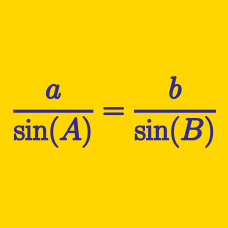Geometry

# Extended Sine Rule

In triangle $ABC$, let $a, b$ and $c$ be the lengths of the legs of a triangle opposite vertices $A, B, C$ respectively. Let $\alpha, \beta, \gamma$ be the values of the respective angles. What is the value of

$a ( \sin \beta - \sin \gamma) + b ( \sin \gamma - \sin \alpha ) + c ( \sin \alpha - \sin \beta )?$

Triangle $ABC$ is inscribed in a circle $\Gamma$. If $\angle A = 60^{\circ}$ and the length of the side opposite to vertex $A$ is $49 \sqrt{3}$, what is the radius of $\Gamma$?

In triangle $ABC$, $\angle ABC = \frac{ \pi}{2}$. If $AC = 12$, what is the radius of the circumcicle of $ABC$?

Triangle $ABC$ has $\angle A=150^{\circ}$ and $\overline{BC}=7$. If the area of the circumscribed circle of $ABC$ is $a\pi$, what is the value of $a$?

Triangle $ABC$ satisfies $2\sin B=\sin A+\sin C$. If $a+c=30$, what is the value of $b$?

Details and assumptions

$a$, $b$ and $c$ are the lengths of the sides opposite to the vertices $A$, $B$ and $C$, respectively.

×